# Price Elasticity of Demand

Price Elasticity is defined as the percentage change in the quantity of a particular item demanded with the unit percentage change in the price of that item. Price elasticity is represented by (PED or Ed).
• It is obtained by dividing the percentage change in quantity (∆D/D) by percentage change in price (∆P/P).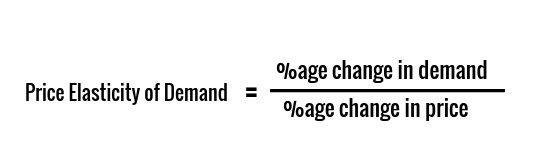### Examples on price elasticity calculation:

#### Example 1:

• Suppose the price of sugar was Rs 75/kg on January 21, 2020, which increased to Rs 80/kg on February 21, 2020. Now, let us take the daily demand of sugar which came down from 1000 kg on January 21, 2020, to 980 kg on February 21, 2020. Calculate the price elasticity of demand for petrol based on the given information.
• % change in demand = (980 - 1000)/1000 = (-)2%
• % change in price = (80 - 75)/75= 6.67%
• So, Price elasticity of demand (PED) = (-)2/6.67 = (-)0.3

#### Example 2:

• The price of ice cream decreased from Rs 20/scoop to Rs 22/scoop. If the demand for ice cream increases from 10 scoops to 1 scoop. Calculate the price elasticity of demand for ice cream based on the given information.
• % change in demand = (1 - 10)/10 = (-) 90%
• % change in price = (22 - 20)/20= 10%
• So, Price elasticity of demand (PED) = (-)90/10 = (-)9

• The Law of Demand suggests that demand for goods moves in the opposite direction of its price. So, PED comes out to be negative for normal goods. But the impact of price change is not the same in all the cases. For some goods, demand changes considerably even for small price changes. On the other hand, there are certain goods whose demand is not affected much by price changes.
• In the case of Example 1, the demand for sugar has not changed considerably with a change in price. This is because items like sugar, salt, rice, wheat, etc. are essential items. People consume them irrespective of their price. These items for which demand does not change considerably with a change in price are known as Inelastic. For Inelastic goods, the magnitude of PED is always less than 1.
• In the case of Example 2, the demand for ice cream has changed considerably with little change in price. This is because items like ice cream, pizza, air conditioners, cars, etc. are luxury items. People buy them when they can afford it. These items for which demand changes considerably with little change in price are known as Elastic. For Elastic goods, the magnitude of PED is always greater than 1.

## Some Examples of Elastic Goods:

• Coffee
• Airline Tickets
• Stocks/Shares of a Company
• Ice cream
• Housing
• Cellphones
• Vacations
• Jewelry
• Automobiles
• Clothes, Shoes, and accessories, etc.

## Some Examples of Inelastic Goods:

• Daily use Food products (Milk, Salt, rice, wheat, sugar, etc.)
• Lifesaving Medicines
• Electricity
• Fuel
• Addictive things like drugs and cigarettes, etc.

## Types of Elasticity (Based on the magnitude of PED values)

• Based on the values of |PED|, demands can be of the following five types:
• Perfectly Inelastic
• Relatively Inelastic
• Unit Elastic
• Relatively Elastic
• Perfectly Inelastic

### Perfectly Inelastic:

• The items for which demand does not change at all with a change in price. Since the change in demand is zero, the PED comes out to be zero.• For Perfectly Inelastic, |PED| = 0
• This is an ideal concept and there are no examples of perfectly inelastic goods. Because if this was the case, producers would be able to charge whatever they felt like and consumers would still need to buy them.
• The only examples of perfectly inelastic items can be air and water. But they are free goods as they are not charged.
• The demand curve for Perfectly Inelastic is a vertical line because the quantity demanded is the same at any price

#### Demand Curve for Perfectly Inelastic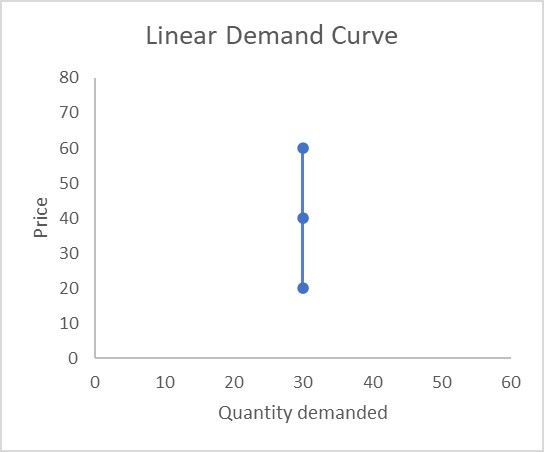### Relatively Inelastic:

• The items for which percentage change in quantity demanded is less than the percentage change in price, hence the |PED| comes out to be less than 1.• For Relatively Inelastic, |PED| < 1
• Examples of relatively inelastic goods are Daily use of Food products, Lifesaving Medicines, Electricity, Fuel, etc.
• In the monopoly and oligopoly market, items are relatively inelastic.
• The demand curve for Relatively Inelastic is steeper and the slope will be more because the change in quantity demanded is less than a change in price.

#### Demand Curve for Relatively Inelastic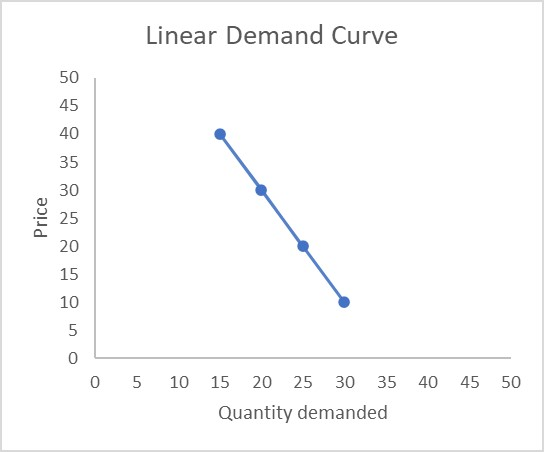### Unit elastic:

• The items for which a change in price will cause an equal proportional change in quantity demanded. The percentage change in demand is the same as the percentage change in the price of the item, hence |PED| comes out to be 1.• For Unit Elastic, |PED| = 1

#### Example 3:

• The price of a laptop was $1000 on January 21, 2020, which increased to$ 2000 on February 21, 2020. This led to a decrease in sales of laptops from 5000 on January 21, 2020, to 2500 on February 21, 2020. Calculate the price elasticity of demand for petrol based on the given information. Also, determine if the demand for a laptop is unit elastic.
• % change in demand = (2500 - 5000)/5000 = (-)50%
• % change in price = (2000 - 1000)/1000 = 50%
• So, Price elasticity of demand (PED) = (-)50/50 = (-)1
• Since |PED| = 1, the demand is unit elastic

#### Demand Curve for Unit Elastic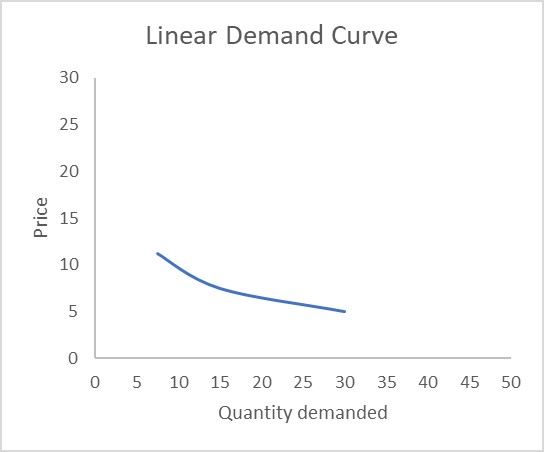### Relatively Elastic:

• The items for which percentage change in quantity demanded is greater than the percentage change in price, hence the |PED| comes out to be more than 1.• For Relatively elastic, |PED| > 1
• Examples of relatively elastic goods are Coffee, Airline Tickets, Stocks/Shares of a Company, Ice cream, Housing
• In the perfect competition market, demands are relatively elastic.
• The demands of goods for which close substitutes are available are also relatively elastic.
• The demand curve for Relatively elastic is flatter and the slope will be less because the change in quantity demanded is less than a change in price.

#### Demand Curve for Relatively Elastic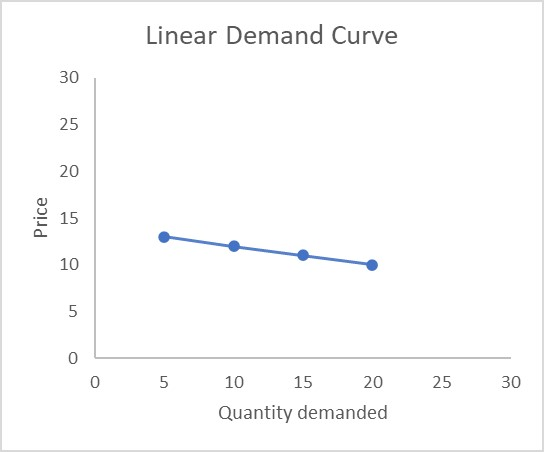### Perfectly Elastic:

• The items for which even a small change in price causes an infinite change in the quantity demanded. Since the change in quantity demanded is infinite, |PED| comes out to be infinity.• For Perfectly elastic, |PED| = ∞
• This is an ideal concept and there are no examples of perfectly elastic goods.
• The examples which are close to perfectly elastic items are:
1. Vegetable/ Fruit Vendors located on a street. - Here all the vendors have to keep almost the same price to get the demand; if any of them would increase their price people would tend to move to another vendor. So, such a vendor faces a perfectly elastic demand curve.
2. Currency Exchange Market - If someone is offering a lower price for your currency (i.e. a higher price to you) you would go to some other firm to sell off your currency. And the first one would lose its market share.
• The demand curve for Perfectly Elastic is a straight horizontal line which shows that the market demand for a product is directly tied to the price.

#### Demand Curve for Perfectly Elastic